x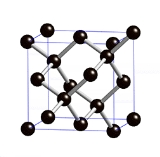Diamond cubicOverview

The diamond cubic crystal structure
Crystal structure
In mineralogy and crystallography, crystal structure is a unique arrangement of atoms or molecules in a crystalline liquid or solid. A crystal structure is composed of a pattern, a set of atoms arranged in a particular way, and a lattice exhibiting long-range order and symmetry...

is a repeating pattern of 8 atoms that certain material
Material
Material is anything made of matter, constituted of one or more substances. Wood, cement, hydrogen, air and water are all examples of materials. Sometimes the term "material" is used more narrowly to refer to substances or components with certain physical properties that are used as inputs to...

s may adopt as they solidify. While the first known example was diamond
Diamond
In mineralogy, diamond is an allotrope of carbon, where the carbon atoms are arranged in a variation of the face-centered cubic crystal structure called a diamond lattice. Diamond is less stable than graphite, but the conversion rate from diamond to graphite is negligible at ambient conditions...

, other elements in group IV
Carbon group
The carbon group is a periodic table group consisting of carbon , silicon , germanium , tin , lead , and ununquadium ....

also adopt this structure, including tin
Tin
Tin is a chemical element with the symbol Sn and atomic number 50. It is a main group metal in group 14 of the periodic table. Tin shows chemical similarity to both neighboring group 14 elements, germanium and lead and has two possible oxidation states, +2 and the slightly more stable +4...

, the semiconductor
Semiconductor
A semiconductor is a material with electrical conductivity due to electron flow intermediate in magnitude between that of a conductor and an insulator. This means a conductivity roughly in the range of 103 to 10−8 siemens per centimeter...

s silicon
Silicon
Silicon is a chemical element with the symbol Si and atomic number 14. A tetravalent metalloid, it is less reactive than its chemical analog carbon, the nonmetal directly above it in the periodic table, but more reactive than germanium, the metalloid directly below it in the table...

and germanium
Germanium
Germanium is a chemical element with the symbol Ge and atomic number 32. It is a lustrous, hard, grayish-white metalloid in the carbon group, chemically similar to its group neighbors tin and silicon. The isolated element is a semiconductor, with an appearance most similar to elemental silicon....

, and silicon/germanium alloy
Alloy
An alloy is a mixture or metallic solid solution composed of two or more elements. Complete solid solution alloys give single solid phase microstructure, while partial solutions give two or more phases that may or may not be homogeneous in distribution, depending on thermal history...

s in any proportion.
Diamond cubic is in the Fdm space group
Space group
In mathematics and geometry, a space group is a symmetry group, usually for three dimensions, that divides space into discrete repeatable domains.In three dimensions, there are 219 unique types, or counted as 230 if chiral copies are considered distinct...

, which follows the face-centered cubic
Cubic crystal system
In crystallography, the cubic crystal system is a crystal system where the unit cell is in the shape of a cube. This is one of the most common and simplest shapes found in crystals and minerals....

bravais lattice.DiscussionsMaterial scienceDiamond cubic structureEncyclopedia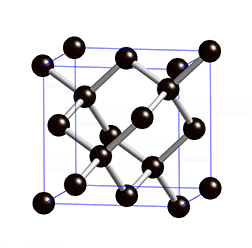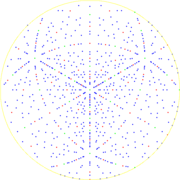The diamond cubic crystal structure
Crystal structure
In mineralogy and crystallography, crystal structure is a unique arrangement of atoms or molecules in a crystalline liquid or solid. A crystal structure is composed of a pattern, a set of atoms arranged in a particular way, and a lattice exhibiting long-range order and symmetry...

is a repeating pattern of 8 atoms that certain material
Material
Material is anything made of matter, constituted of one or more substances. Wood, cement, hydrogen, air and water are all examples of materials. Sometimes the term "material" is used more narrowly to refer to substances or components with certain physical properties that are used as inputs to...

s may adopt as they solidify. While the first known example was diamond
Diamond
In mineralogy, diamond is an allotrope of carbon, where the carbon atoms are arranged in a variation of the face-centered cubic crystal structure called a diamond lattice. Diamond is less stable than graphite, but the conversion rate from diamond to graphite is negligible at ambient conditions...

, other elements in group IV
Carbon group
The carbon group is a periodic table group consisting of carbon , silicon , germanium , tin , lead , and ununquadium ....

also adopt this structure, including tin
Tin
Tin is a chemical element with the symbol Sn and atomic number 50. It is a main group metal in group 14 of the periodic table. Tin shows chemical similarity to both neighboring group 14 elements, germanium and lead and has two possible oxidation states, +2 and the slightly more stable +4...

, the semiconductor
Semiconductor
A semiconductor is a material with electrical conductivity due to electron flow intermediate in magnitude between that of a conductor and an insulator. This means a conductivity roughly in the range of 103 to 10−8 siemens per centimeter...

s silicon
Silicon
Silicon is a chemical element with the symbol Si and atomic number 14. A tetravalent metalloid, it is less reactive than its chemical analog carbon, the nonmetal directly above it in the periodic table, but more reactive than germanium, the metalloid directly below it in the table...

and germanium
Germanium
Germanium is a chemical element with the symbol Ge and atomic number 32. It is a lustrous, hard, grayish-white metalloid in the carbon group, chemically similar to its group neighbors tin and silicon. The isolated element is a semiconductor, with an appearance most similar to elemental silicon....

, and silicon/germanium alloy
Alloy
An alloy is a mixture or metallic solid solution composed of two or more elements. Complete solid solution alloys give single solid phase microstructure, while partial solutions give two or more phases that may or may not be homogeneous in distribution, depending on thermal history...

s in any proportion.

## Crystallographic structure

Diamond cubic is in the Fdm space group
Space group
In mathematics and geometry, a space group is a symmetry group, usually for three dimensions, that divides space into discrete repeatable domains.In three dimensions, there are 219 unique types, or counted as 230 if chiral copies are considered distinct...

, which follows the face-centered cubic
Cubic crystal system
In crystallography, the cubic crystal system is a crystal system where the unit cell is in the shape of a cube. This is one of the most common and simplest shapes found in crystals and minerals....

bravais lattice. The lattice describes the repeat pattern; for diamond cubic crystals this lattice is "decorated" with a motif of two tetrahedrally
Tetrahedron
In geometry, a tetrahedron is a polyhedron composed of four triangular faces, three of which meet at each vertex. A regular tetrahedron is one in which the four triangles are regular, or "equilateral", and is one of the Platonic solids...

bonded
Chemical bond
A chemical bond is an attraction between atoms that allows the formation of chemical substances that contain two or more atoms. The bond is caused by the electromagnetic force attraction between opposite charges, either between electrons and nuclei, or as the result of a dipole attraction...

atoms in each primitive cell
Wigner–Seitz cell
The Wigner–Seitz cell, named after Eugene Wigner and Frederick Seitz, is a type of Voronoi cell used in the study of crystalline material in solid-state physics....

, separated by 1/4 of the width of the unit cell in each dimension. Many compound semiconductor
Compound semiconductor
A compound semiconductor is a semiconductor compound composed of elements from two or more different groups of the periodic table . These semiconductors typically form in groups 13-16 ,...

s such as gallium arsenide, β-silicon carbide
Silicon carbide
Silicon carbide , also known as carborundum, is a compound of silicon and carbon with chemical formula SiC. It occurs in nature as the extremely rare mineral moissanite. Silicon carbide powder has been mass-produced since 1893 for use as an abrasive...

and indium antimonide adopt the analogous zincblende structure, where each atom has nearest neighbors of an unlike element. Zincblende's space group is F3m, but many of its structural properties are quite similar to the diamond structure.

The atomic packing factor
Atomic packing factor
In crystallography, atomic packing factor or packing fraction is the fraction of volume in a crystal structure that is occupied by atoms. It is dimensionless and always less than unity. For practical purposes, the APF of a crystal structure is determined by assuming that atoms are rigid spheres...

of the diamond cubic structure (the proportion of space that would be filled by spheres that are centered on the vertices of the structure and are as large as possible without overlapping) is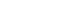, significantly smaller (indicating a less dense structure) than the packing factors for the face-centered and body-centered cubic lattices
Cubic crystal system
In crystallography, the cubic crystal system is a crystal system where the unit cell is in the shape of a cube. This is one of the most common and simplest shapes found in crystals and minerals....

. Zinc blende structures have higher packing factors than 0.34 depending on the relative sizes of their two component atoms.

The first, second, third and fourth nearest-neighbor distances in units of the cubic lattice constant are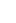,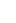,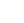, and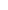, respectively.

## Mathematical structure

Mathematically, the points of the diamond cubic structure can be given coordinates as a subset of a three-dimensional integer lattice
Integer lattice
In mathematics, the n-dimensional integer lattice , denoted Zn, is the lattice in the Euclidean space Rn whose lattice points are n-tuples of integers. The two-dimensional integer lattice is also called the square lattice, or grid lattice. Zn is the simplest example of a root lattice...

by using a cubical unit cell four units across. With these coordinates, the points of the structure have coordinates (xyz) satisfying the equations
x = y = z (mod 2), and
x + y + z = 0 or 1 (mod 4).

There are eight points (modulo 4) that satisfy these conditions:, (0,2,2), (2,0,2), (2,2,0),, (3,1,1), (1,3,1), (1,1,3)
All of the other points in the structure may be obtained by adding multiples of four to the x, y, and z coordinates of these eight points. Adjacent points in this structure are at distance √3 apart in the integer lattice; the edges of the diamond structure lie along the body diagonals of the integer grid cubes. This structure may be scaled to a cubical unit cell that is some number a of units across by multiplying all coordinates by a/4.

Alternatively, each point of the diamond cubic structure may be given four-dimensional integer coordinates that sum to either zero or one. Two points are adjacent in the diamond structure if and only if their four-dimensional coordinates differ by one in a single coordinate. The total difference in coordinate values between any two points (their four-dimensional Manhattan distance) gives the number of edges in the shortest path between them in the diamond structure. The four nearest neighbors of each point may be obtained, in this coordinate system, by adding one to each of the four coordinates, or by subtracting one from each of the four coordinates, accordingly as the coordinate sum is zero or one. These four-dimensional coordinates may be transformed into three-dimensional coordinates by the formula → (a + bcd, ab + cd, −a + b + cd).
Because the diamond structure forms a distance-preserving
Isometry
In mathematics, an isometry is a distance-preserving map between metric spaces. Geometric figures which can be related by an isometry are called congruent.Isometries are often used in constructions where one space is embedded in another space...

subset of the four-dimensional integer lattice, it is a partial cube
Partial cube
In graph theory, a partial cube is a graph that is an isometric subgraph of a hypercube. In other words, a partial cube is a subgraph of a hypercube that preserves distances—the distance between any two vertices in the subgraph is the same as the distance between those vertices in the hypercube...

.

Yet another coordinatization of the diamond cubic involves the removal of some of the edges from a three-dimensional grid graph. In this coordinatization, which has a distorted geometry from the standard diamond cubic structure but has the same topological structure, the vertices of the diamond cubic are represented by all possible 3d grid points and the edges of the diamond cubic are represented by a subset of the 3d grid edges.

The diamond cubic is sometimes called the "diamond lattice" but it is not, mathematically, a lattice
Lattice (group)
In mathematics, especially in geometry and group theory, a lattice in Rn is a discrete subgroup of Rn which spans the real vector space Rn. Every lattice in Rn can be generated from a basis for the vector space by forming all linear combinations with integer coefficients...

: there is no translational symmetry
Translational symmetry
In geometry, a translation "slides" an object by a a: Ta = p + a.In physics and mathematics, continuous translational symmetry is the invariance of a system of equations under any translation...

that takes the point (0,0,0) into the point (3,3,3), for instance. However, it is still a highly symmetric structure: any incident pair of a vertex and edge can be transformed into any other incident pair by a congruence
Congruence (geometry)
In geometry, two figures are congruent if they have the same shape and size. This means that either object can be repositioned so as to coincide precisely with the other object...

of Euclidean space
Euclidean space
In mathematics, Euclidean space is the Euclidean plane and three-dimensional space of Euclidean geometry, as well as the generalizations of these notions to higher dimensions...

.

## Manufacturing considerations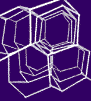Since materials with diamond or zinc blende structures are important for formation of wafers
Wafer (electronics)
A wafer is a thin slice of semiconductor material, such as a silicon crystal, used in the fabrication of integrated circuits and other microdevices...

used in the fabrication of modern electronics
Electronics
Electronics is the branch of science, engineering and technology that deals with electrical circuits involving active electrical components such as vacuum tubes, transistors, diodes and integrated circuits, and associated passive interconnection technologies...

, it is important to know that they present open, hexagonal ion channel
Ion channel
Ion channels are pore-forming proteins that help establish and control the small voltage gradient across the plasma membrane of cells by allowing the flow of ions down their electrochemical gradient. They are present in the membranes that surround all biological cells...

s when ion implantation
Ion implantation
Ion implantation is a materials engineering process by which ions of a material are accelerated in an electrical field and impacted into another solid. This process is used to change the physical, chemical, or electrical properties of the solid...

is carried out from any of the <110> directions (that is, 45° from one of the cube edges). Their open structure also results in a volume reduction upon melting or amorphization
Amorphous solid
In condensed matter physics, an amorphous or non-crystalline solid is a solid that lacks the long-range order characteristic of a crystal....

, as is also seen in ice
Ice
Ice is water frozen into the solid state. Usually ice is the phase known as ice Ih, which is the most abundant of the varying solid phases on the Earth's surface. It can appear transparent or opaque bluish-white color, depending on the presence of impurities or air inclusions...

.

They display octahedral
Octahedron
In geometry, an octahedron is a polyhedron with eight faces. A regular octahedron is a Platonic solid composed of eight equilateral triangles, four of which meet at each vertex....

cleavage
Cleavage (crystal)
Cleavage, in mineralogy, is the tendency of crystalline materials to split along definite crystallographic structural planes. These planes of relative weakness are a result of the regular locations of atoms and ions in the crystal, which create smooth repeating surfaces that are visible both in the...

, which means that they have four planes—directions following the faces of the octahedron where there are fewer bonds
Chemical bond
A chemical bond is an attraction between atoms that allows the formation of chemical substances that contain two or more atoms. The bond is caused by the electromagnetic force attraction between opposite charges, either between electrons and nuclei, or as the result of a dipole attraction...

and therefore points of structural weakness—along which single crystals can easily split, leaving smooth surfaces. Similarly, this lack of bonds can guide chemical etching of the right chemistry (i.e., potassium hydroxide
Potassium hydroxide
Potassium hydroxide is an inorganic compound with the formula KOH, commonly called caustic potash.Along with sodium hydroxide , this colorless solid is a prototypical strong base. It has many industrial and niche applications. Most applications exploit its reactivity toward acids and its corrosive...

solutions for Si) to produce pyramidal structures such as mesas, points, or etch pits, a useful technique for microelectromechanical systems
Microelectromechanical systems
Microelectromechanical systems is the technology of very small mechanical devices driven by electricity; it merges at the nano-scale into nanoelectromechanical systems and nanotechnology...

.